# ODEnetwork

## Introduction

Simulating a network of (damped) harmonic oscillators as described in Wikipedia over time. A connection between two oscillators consists of a damper and a spring. It is possible to insert events over time.

## Simulation of a Single Oscillator

Our task is the simulation of a single harmonic oscillator.

### Definition

We define an oscillator with a mass which is connected to a spring and a damper.

mass = 1
damper = matrix(0.1)
spring = matrix(1)
odenet = ODEnetwork(masses = mass, dampers = damper, springs = spring)

In the next step, it is necessary to define the start conditions.

position = 1
velocity = 0
odenet = setState(odenet = odenet, state1 = position, state2 = velocity)

### Results

Finally, we run the simulation over a time interval of 20 seconds and plot the result of the first state, which is the position of the oscillator.

odenet = simuNetwork(odenet = odenet, times = seq(0, 20))
plot(odenet, state = "1")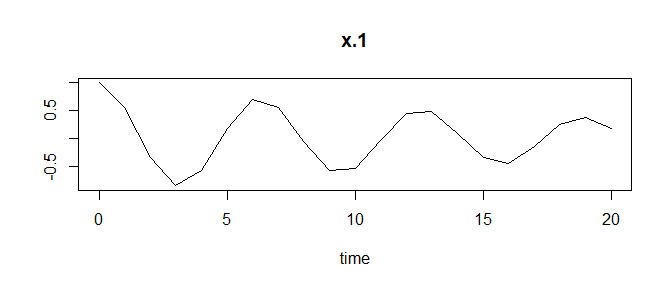This looks a bit ugly, because ODEnetwork calculates the position (state1) of the oscillator for the times given in vector times. The calculation for every time stamp is correct, because the solution is calculated in an analytic way:

odenet$simulation$method
#>  "analytic"

We can improve the smoothness by using a time vector with smaller steps.

odenet = simuNetwork(odenet = odenet, times = seq(0, 20, 0.01))
plot(odenet, state = "1")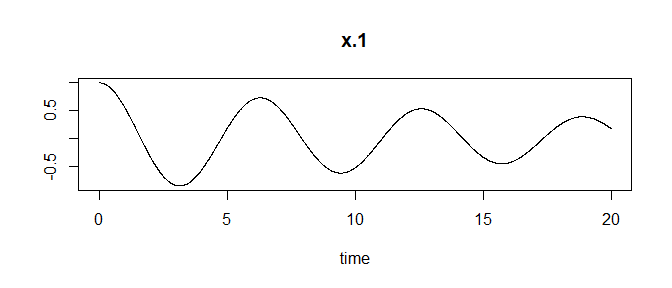## Simulation of a Network

Complexer questions handle connected oscillators instead of one. It is interesting, to simulate the behaviour of each oscillator connected to the others in a network of oscillators. Here we take a closer look at three oscillators which are connected in a row. That means, oscillator 1 is connected with oscillator 2, which is connected to oscillator 3.

### Definition

As in the section above, we define the masses, dampers, and springs. Additionally, it is necessary to define connections between the oscillators. The corresponding values are set to the off-diagonal elements in the variables damper and spring.

mass = 1:3
damper = diag(rep(0.1, 3))
damper[1, 2] = 0.1
damper[2, 3] = 0.1
spring = diag(rep(1, 3))
spring[1, 2] = 1
spring[2, 3] = 1
odenet = ODEnetwork(masses = mass, dampers = damper, springs = spring)

In the next step, it is necessary to define the start conditions for the two states which are the position and velocity of each oscillator.

position = rep(1, 3)
velocity = c(0, 1, -1)
odenet = setState(odenet = odenet, state1 = position, state2 = velocity)

### Results

#### Graphs

Finally, we run the simulation over a time interval of 20 seconds and plot the result of the first state, which is the position of the oscillator.

odenet = simuNetwork(odenet = odenet, times = seq(0, 20, 0.01))
plot(odenet, state = "1")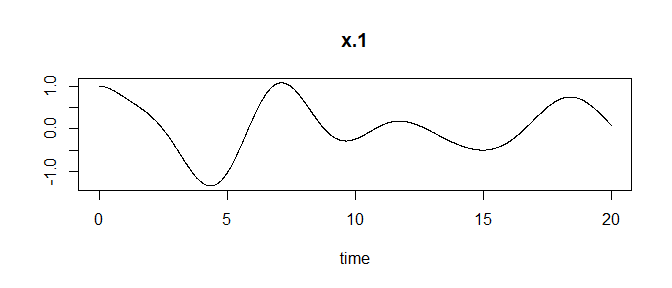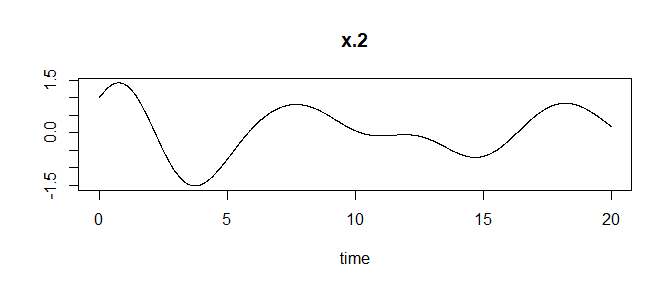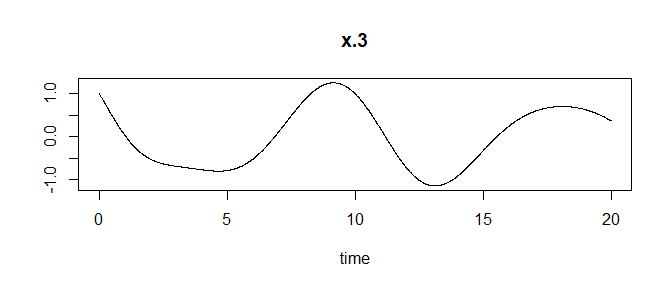It is possible to take a look at the position x.1 and velocity v.1 in one graph:

plot(odenet, state = "1vs2", var = 1)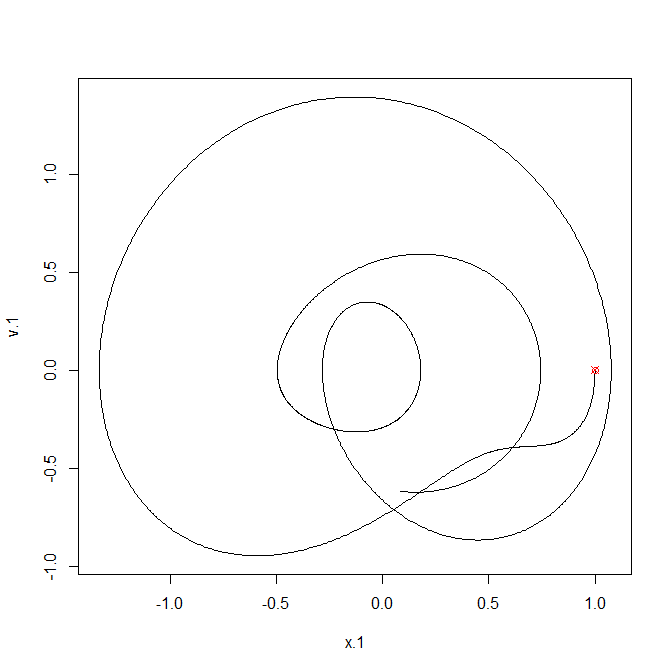#### Calculations

To calculate the resonance frequencies of each oscillator we call the function calcResonances().

calcResonances(odenet)
#>   cResonances
#> 1  0.15915494
#> 2  0.11253954
#> 3  0.09188815

By default all springs in these networks have the length 0. Using the variable distances in ODEnetwork or updateOscillators we can define values for the spring length. Sometimes it is necessary to calculate these distances from an equilibrium state. So, what would be a possible set of spring length, if we provide an equilibrium state? We can calculate this via the function estimateDistances().

odenet = estimateDistances(odenet, equilibrium = c(1, 2, 3))
odenet$distances #> [,1] [,2] [,3] #> [1,] 2.000000 1.555628 0.000000 #> [2,] 1.555628 2.000000 1.555628 #> [3,] 0.000000 1.555628 2.000000 odenet = estimateDistances(odenet, equilibrium = c(1, 2, 3), distGround = "individual") odenet$distances
#>           [,1]      [,2]      [,3]
#> [1,] 0.3116121 0.6882909 0.0000000
#> [2,] 0.6882909 1.9998933 0.6886108
#> [3,] 0.0000000 0.6886108 3.6884944

The first calculation defines the spring length from the ground (or reference point) equally. It estimates the spring length between the oscillators with one value, because all spring constants are equal. The second calculation enables the estimation to calculate individual length to the reference point, which effects the distances between the oscillators, too.

## Simulation with Events over Time

All calculations in the examples above are done from a defined starting point over time in an analytical way. In some applications it is interesting to interact with the oscillators during the simulation. The following example uses two connected oscillators.

mass = c(1, 2)
damper = diag(c(0.02, 0.1))
damper[1, 2] = 0.1
spring =  diag(c(4, 1))
spring[1, 2] = 2
distance = matrix(c(0, 0, 1, 0), ncol = 2)
odenet = ODEnetwork(mass, damper, spring, distances = distance)
odenet = setState(odenet, c(1, 1), c(0, 0))
odenet = simuNetwork(odenet, seq(0, 20, by = 0.01))

Here we see the positions over time without interactions during the simulation.

plot(odenet, state = "1")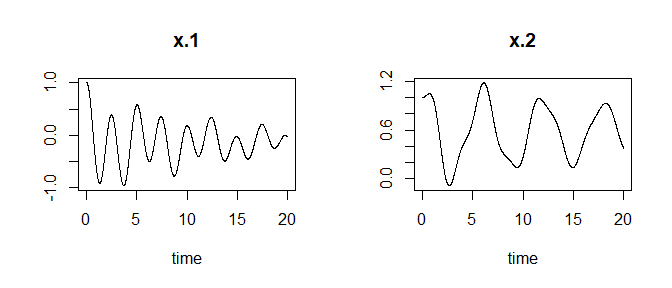In the next step, we define some events and assign the to the network. The following data.frame defines events for the position of the first oscillator. Using the setEvents method, we assign the events to the network. The type parameter is set to linear, hence the coordinates defined by time and value are connected using a linear function. We see the effect of this parameter in the next plot. After setting everything, the simulation has to be recalculated again.

eventdata = data.frame(var = c("x.1", "x.1", "x.1")
, time = c(5, 10, 12)
, value = c(0, 1, 1)
, stringsAsFactors = TRUE
)
odenet = setEvents(odenet, eventdata, type = "constant")
odenet = simuNetwork(odenet, seq(0, 20, by = 0.01))
plot(odenet, state = "1")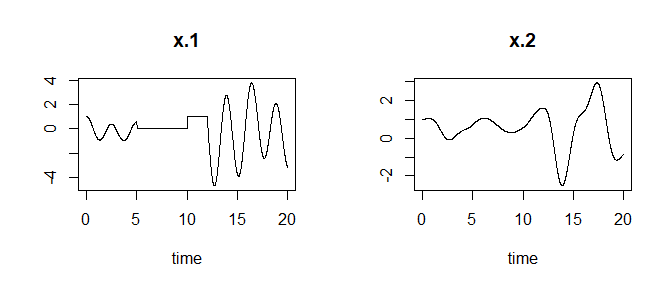As we see in the plot, the position of the oscillator is set to 0 at time 5 seconds. After 10 seconds the position is set to 1 and the oscillator changes its position in a linear way. Change the values of the parameter type to dirac or constant to get an idea of their different behaviour.

These calculations are done in a numeric way. We see this in the following variable of odenet which describes the used calculation method.

odenet$simulation$method
#>  "lsoda"